Refer to our Texas Go Math Grade 3 Answer Key Pdf to score good marks in the exams. Test yourself by practicing the problems from Texas Go Math Grade 3 Lesson 19.2 Answer Key Pictographs.

Unlock the Problem

A pictograph is a graph that uses pictures or symbols to show and compare the information.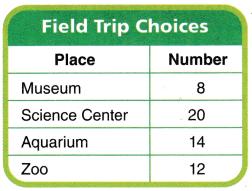Delia made the table at the right. She used it to record the places the third grade classes would like to go during a field trip. How can you show the data in a pictograph?

Make a pictograph

STEP 1:
Write the title. Write the name of a place in each row.

STEP 2:
Choose a picture for the key, and let each picture represent 4 students.

STEP 3:
Draw the correct number of pictures for each field trip choice.Math idea
A key is a part of a graph that explains the symbols.
In this graph,
= 4 students,
so = 2 students.

How did you decide how many pictures to draw for Aquarium?Math idea
A key is a part of a graph that explains the symbols.
In this graph,= 4 students,   so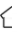= 2 students.
Explanation:
Done the pictograph with the given information

Try This!

Make a pictograph from data you collect. Take a survey or observe a subject that interests you. Collect and record the data in a frequency table. Then make a pictograph. Decide on a symbol and a key. Include a title and labels.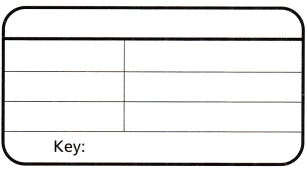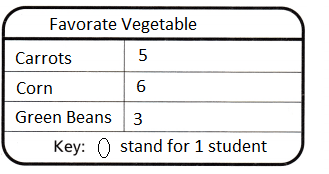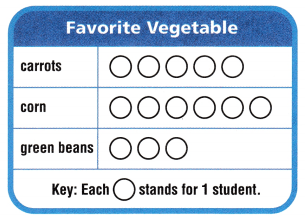Explanation:
Done a pictograph from data I collect.
Taken the survey and observed a subject that interests me. Collected and recorded the data in a frequency table. Then Done a pictograph. Decided on a symbol and a key.

Share and Show

Jeremy pulled marbles from a bag one at a time, recorded their color, and then put them back in the bag. Make a pictograph of the data. Use this key:
Each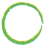2 = marbles.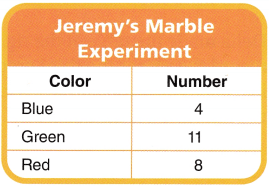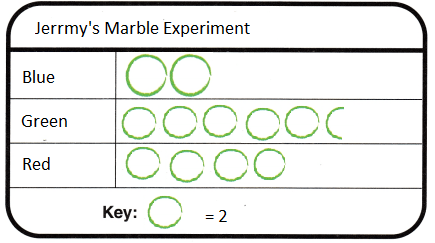Explanation:
Completed the Pictograph with the help of Frequency table
Use your pictograph above for 1-2.
Question 1.
How many more times did Jeremy pull out a red marble than a blue marble?
Explanation:
red marbles =  8
Blue marbles = 4
8 – 4 = 4
4 more times did Jeremy pull out a red marble than a blue marble

Question 2.
How many fewer times did Jeremy pull out green marbles than blue and red marbles combined?
Red marbles = 8
Green marbles= 11
blue = 4
red and blue combined 8 + 4 = 12
12 – 11 = 1
Explanation:
1 fewer times  Jeremy pull out green marbles than blue and red marbles combined

Math Talk
Mathematical Processes

Explain how you knew how many pictures to draw for green.
Answer:= 2
Explanation:
so, drawn 5 full and a half
in all shows 11
Then half of it is equal to 1

Problem Solving

Question 3.
Representations While at the Science Center, Delia’s classmates learned how many teeth some mammals have. Use the data in the table to make a pictograph. Use this key: Each △ = 4 teeth.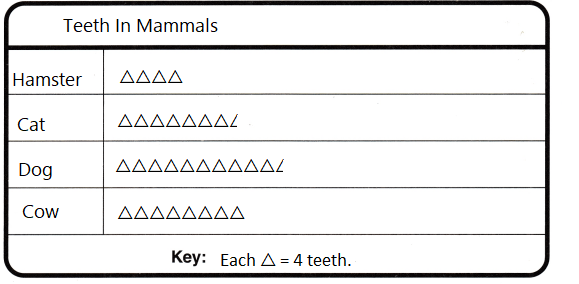Use your pictograph above for 4-7.

Question 4.
Analyze How many more teeth do cows have than hamsters?
Explanation:
16 more teeth do cows have than hamsters
cows have 32
hamsters have 16
32 – 16 = 32

Question 5.
H.O.T. Pose a Problem Write a problem that can be solved by using the data in your pictograph. Then solve the problem.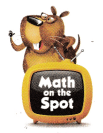Question 6.
H.O.T. Multi-Step How many fewer teeth do cats and hamsters have combined than dogs and cows combined?
cats and hamsters have 46
dogs and cows have 74
38 fewer teeth do cats and hamsters have combined than dogs and cows combined

Question 7.
Use Symbols How many pictures would you draw for Cat if each △ = 5 teeth?
Explanation:
Cat has 30 teeth
6 x 5 = 30,  △ = 5 teeth

Fill in the bubble for the correct answer choice.

Use the Balloon Animals Made on Sunday pictograph for 8-11.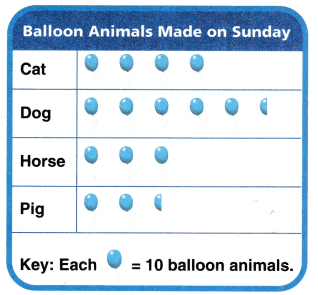Question 8.
Analyze Landon made balloon animals in the park on Sunday. How many dog balloon animals did Landon make?
(A) 5
(B) 55
(C) 50
(D) 25
Explanation:
Landon made balloon animals in the park on Sunday
55 dog balloon animals made by Landon
5 x 10 + half of a balloon = 50 + 5 = 55

Question 9.
Multi-Step How many balloon animals did Landon make in all on Sunday?
(A) 15
(B) 140
(C) 150
(D) 160
Explanation:
In total there are 14 + 2 half balloons
1 balloon = 10
15 x 10 = 150

Question 10.
Multi-Step On Monday, Landon made 15 fewer pig balloon animals than he made on Sunday. What would a pictograph for the balloon animals made on Monday show for pig balloon animals?Explanation:
On Monday, Landon made 15 fewer pig balloon animals than he made on Sunday.
Number of pig balloons made on Sunday are 25
25 – 15 = 10
1 balloon = 10

Texas Test Prep

Question 11.
Representations How many pictures would you draw for Cat if each= 5 balloon animals?
(A) 6
(B) 8
(C) 5
(D) 2
Explanation:
8are drawn if  theis equal to 5
cat = 40
8 x 5 = 40

### Texas Go Math Grade 3 Lesson 19.2 Homework and Practice Answer Key

Problem Solving

Question 1.
During recycling day, teams of students collected the materials shown in the table. Use the data in the table to make a pictograph. Use this key:
Each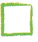= 4 materials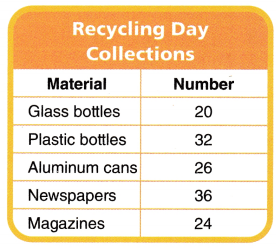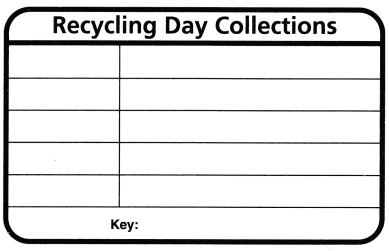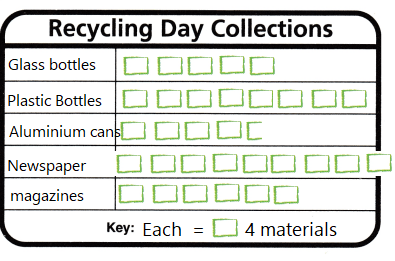Explanation:
Completed the Pictograph with the help of Frequency table

Question 2.
How many more plastic bottles were collected than glass bottles?
Explanation:
Number of plastic bottles = 32
Number of glass bottles = 20
32 – 20 = 12
12 more plastic bottles were collected than glass bottles

Question 3.
How many more newspapers were collected than magazines?
Explanation:
Number of newspaper 36
number of magazines 24
36-24 = 12
12 more newspapers were collected than magazines

Question 4.
How many newspapers and magazines were collected?
Explanation:
Number of newspaper 36
number of magazines 24
36 +24 = 60
60  newspapers and magazines were collected

Question 5.
How many plastic and glass bottles were collected?
Explanation:
Number of plastic bottles = 32
Number of glass bottles = 20
32 + 20 = 52
52 plastic and glass bottles were collected

Lesson Check

Use the Animals on the Farm pictograph for 6-8.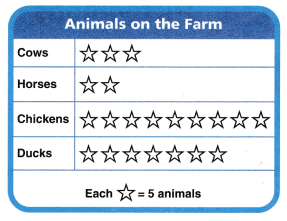Question 6.
Multi-Step The pictograph shows the number of animals at Mr. DiPiero’s farm. How many more chickens than ducks does Mr. DiPiero have?
(A) 3
(B) 15
(C) 10
(D) 16
Explanation:
Number of chickens 9 x 5 = 45
Number of ducks 7 x 5 = 35
45 – 35 = 10

Question 7.
Suppose Mr. DiPiero gets 30 pigs. How many star symbols would he use to show them on the pictograph?
(A) 6
(B) 15
(C) 5
(D) 30
Explanation:
6 x 5 = 30
6 star symbols would he use to show them on the pictograph

Question 8.
Multi-Step How many animals does Mr. DiPiero have on his farm?
(A) 21
(B) 26
(C) 105
(D) 130
Explanation:
Number of chickens 9 x 5 = 45
Number of ducks 7 x 5 = 35
Number of horses 2 x 5 =10
number of cows 3 x 5 = 15
45 + 35 + 10 + 15 = 105

Use Karen’s Jewelry pictograph for 9-11.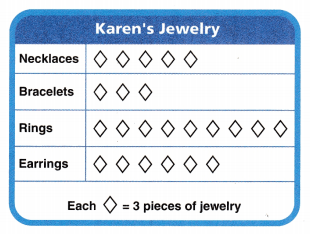Question 9.
Karen has a table at a craft fair to sell jewelry. How many earrings does Karen have to sell?
(A) 27
(B) 18
(C) 3
(D) 6
Explanation:
6 x 3 = 18
Karen has a table at a craft fair to sell jewelry.
18 earrings does Karen have to sell.

Question 10.
Multi-Step Karen sells all of the 11 rings and bracelets. How many pieces of jewelry is this?
(A) 18
(B) 36
(C) 27
(D) 69
Explanation:
number of rings 9 x3 = 27
number of bracelets 3 x 3 = 9
27 + 9 = 36

Question 11.
Multi-Step Karen pairs upsets of necklaces and bracelets. How many necklaces will she have left that do not have matching bracelets?
(A) 6
(B) 2
(C) 5
(D) 12